# Fraction calculator

The calculator performs basic and advanced operations with fractions, expressions with fractions combined with integers, decimals, and mixed numbers. It also shows detailed step-by-step information about the fraction calculation procedure. Solve problems with two, three, or more fractions and numbers in one expression.

## Result:

### (82/3) : 4 = 13/6 = 2 1/6 ≅ 2.1666667

Spelled result in words is thirteen sixths (or two and one sixth).

### How do you solve fractions step by step?

1. Conversion a mixed number 8 2/3 to a improper fraction: 8 2/3 = 8 2/3 = 8 · 3 + 2/3 = 24 + 2/3 = 26/3

To find a new numerator:
a) Multiply the whole number 8 by the denominator 3. Whole number 8 equally 8 * 3/3 = 24/3
b) Add the answer from previous step 24 to the numerator 2. New numerator is 24 + 2 = 26
c) Write a previous answer (new numerator 26) over the denominator 3.

Eight and two thirds is twenty-six thirds
2. Divide: 26/3 : 4 = 26/3 · 1/4 = 26 · 1/3 · 4 = 26/12 = 2 · 13 /2 · 6 = 13/6
Dividing two fractions is the same as multiplying the first fraction by the reciprocal value of the second fraction. The first sub-step is to find the reciprocal (reverse the numerator and denominator, reciprocal of 4/1 is 1/4) of the second fraction. Next, multiply the two numerators. Then, multiply the two denominators. In the following intermediate step, cancel by a common factor of 2 gives 13/6.
In other words - twenty-six thirds divided by four = thirteen sixths.

#### Rules for expressions with fractions:

Fractions - simply use a forward slash between the numerator and denominator, i.e., for five-hundredths, enter 5/100. If you are using mixed numbers, be sure to leave a single space between the whole and fraction part.
The slash separates the numerator (number above a fraction line) and denominator (number below).

Mixed numerals (mixed fractions or mixed numbers) write as integer separated by one space and fraction i.e., 1 2/3 (having the same sign). An example of a negative mixed fraction: -5 1/2.
Because slash is both signs for fraction line and division, we recommended use colon (:) as the operator of division fractions i.e., 1/2 : 3.

Decimals (decimal numbers) enter with a decimal point . and they are automatically converted to fractions - i.e. 1.45.

The colon : and slash / is the symbol of division. Can be used to divide mixed numbers 1 2/3 : 4 3/8 or can be used for write complex fractions i.e. 1/2 : 1/3.
An asterisk * or × is the symbol for multiplication.
Plus + is addition, minus sign - is subtraction and ()[] is mathematical parentheses.
The exponentiation/power symbol is ^ - for example: (7/8-4/5)^2 = (7/8-4/5)2

#### Examples:

subtracting fractions: 2/3 - 1/2
multiplying fractions: 7/8 * 3/9
dividing Fractions: 1/2 : 3/4
exponentiation of fraction: 3/5^3
fractional exponents: 16 ^ 1/2
adding fractions and mixed numbers: 8/5 + 6 2/7
dividing integer and fraction: 5 ÷ 1/2
complex fractions: 5/8 : 2 2/3
decimal to fraction: 0.625
Fraction to Decimal: 1/4
Fraction to Percent: 1/8 %
comparing fractions: 1/4 2/3
multiplying a fraction by a whole number: 6 * 3/4
square root of a fraction: sqrt(1/16)
reducing or simplifying the fraction (simplification) - dividing the numerator and denominator of a fraction by the same non-zero number - equivalent fraction: 4/22
expression with brackets: 1/3 * (1/2 - 3 3/8)
compound fraction: 3/4 of 5/7
fractions multiple: 2/3 of 3/5
divide to find the quotient: 3/5 ÷ 2/3

The calculator follows well-known rules for order of operations. The most common mnemonics for remembering this order of operations are:
PEMDAS - Parentheses, Exponents, Multiplication, Division, Addition, Subtraction.
BEDMAS - Brackets, Exponents, Division, Multiplication, Addition, Subtraction
BODMAS - Brackets, Of or Order, Division, Multiplication, Addition, Subtraction.
GEMDAS - Grouping Symbols - brackets (){}, Exponents, Multiplication, Division, Addition, Subtraction.
Be careful, always do multiplication and division before addition and subtraction. Some operators (+ and -) and (* and /) has the same priority and then must evaluate from left to right.

## Fractions in word problems:

• Find N 2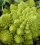Find N = 1 4/5 ÷ 3/5
• Equivalent fractions 2Write the equivalent multiplication expression. 2 1/6÷3/4
• 6/24 change6/24 change to a fraction in lowest terms
• The fourThe four pirates divided 65 coins to each other. They were sorted by age, the youngest receiving the least number of coins, each half more than the previous one. How many coins did the oldest pirate receive?
• Drawing from a hatWhen drawing numbers from a hat from 1 to 35, we select random given numbers. What is the probability that the drawn numbers will be divisible by 8 and 2?
• Sir ToffieOne hot afternoon, Sir Toffie went to his backyard to plant monggo. In his seed box, he found out that different seeds like monggo, pechay, eggplant, and tomatoes were accidentally mixed because of the strong wind. He sieved the mixed seeds and was able t
• Jacob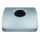Jacob is baking chocolate cookies. The recipe uses 2/8 of a cup of sugar to bake a batch of cookies. If Jacob wants to make one-half of a batch. How many cups of sugar does he need?
• What is 11What is the quotient of Three-fifths and 1 Over 10?
• Nicola 2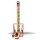Nicola is playing a video game where each round lasts 7/12 of an hour. She has scheduled 3 3/4 hours to play the game. How many rounds can Nicole play?
• JordanJordan wants to bring in cookie cakes to share with his 23 classmates for his birthday. His mom is willing to buy no more than 6 cookie cakes and Jordan must share the cakes equally between his classmates (and himself). Answer the questions to help Jordan
• The balls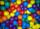You have 108 red and 180 green balls. You have to be grouped into the bags so that the ratio of red to green in each bag was the same. What smallest number of balls may be in one bag?
• Points on line segmentPoints P & Q belong to segment AB. If AB=a, AP = 2PQ = 2QB, find the distance: between point A and the midpoint of the segment QB.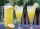Six friends are sharing 8 cups of lemonade. If they share the lemonade equally, how many cups will each friend get?